# Maths Video Lesson 1 Revision eNotes Worksheet and Solutions# Contents

3rd Year Worksheet 1 and Solutions 4

Example 1 & Example 2 4

Example 3 &Example 4 4

Example 5 5

Example 6 &Example 7 5

Example 8 6

Example 9 6

Example 10 7

All revision notes have been produced by mockness ltd for iRevise.com.

Email: info@iRevise.com

All rights reserved; no part of this publication may be reproduced, stored in a retrieval system or transmitted, in any form or by any means, electronic, mechanical, photocopying, recording, reprinting, or otherwise without either the prior written permission of IRevise.com or a license permitting copying in the Republic of Ireland and United Kingdom issued by the copyright licensing Agency.

# 3rd Year Worksheet 1 and Solutions

Now at this stage you should have watched the free Lesson 1 maths tutorial on linear equations and read the iRevise.com lesson 1 revision notes. We are now bringing you a worksheet and solutions.

Example 1 Example 2

Solve 3 ( 2x + 6) = 7 (20 – 10x)

6x + 18 = 140 70x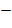6x + 70x = 140 18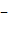76x = 122

x =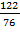= = 1.605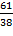Solve 4x 7 = 8 + 2x4x 3x = 8 + 72x = 15

x = = 7.5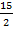Example 3 Example 4

Solve 6(5x) + 3 = 3 2x30 x + 3 = 3 2x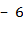33 6x = 3 2x33 + 3 = 2x + 6x36 = 4x

= x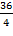9 = x

Solve this rectangle for x.

3x 11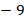5x

5x = 3x 9 + 115x = 3x + 2

5x 3x = 22x = 2

X = = 1## Example 5

Orla will be x years old on her next birthday. Sean will then be 4 years younger than oral.

(i) Write Johns age in terms of x.

(ii) If the sum of their ages is 32, find how old oral will be on her next birthday.

(i) Orla = x (ii) Sum = 32

Sean = x 4 x + x 4 = 322x 4 = 322x = 32 + 4

2x =...